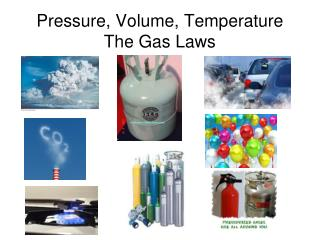DownloadDownload PresentationPressure, Volume, Temperature The Gas Laws

# Pressure, Volume, Temperature The Gas Laws

Télécharger la présentation## Pressure, Volume, Temperature The Gas Laws

- - - - - - - - - - - - - - - - - - - - - - - - - - - E N D - - - - - - - - - - - - - - - - - - - - - - - - - - -
##### Presentation Transcript

1. Pressure, Volume, TemperatureThe Gas Laws

2. Understand the qualitative relationship between pressure (P) and volume (V) and temperature (T), when you have a gas • Learn the three gas laws and how to apply them mathematically to solve problems • Combine the gas laws into one equation, and solve problems where P, V, and T are no longer constant. Learning Objectives

3. Boyle’s Law • Robert Boyle, studied the relationship between pressure, p, and volume, V, in the mid-1600s. • When he doubled the pressure on a sample of gas at constant temperature, its volume was reduced by one-half. pressure volume

4. Boyle’s Law: Graphically • constant temperature • -inverse relationship

5. Connection To Your Math Class Asymptote- A line that continually approaches a given curve but does not meet it at any finite distance. Greek word that means “not falling together”

6. Boyle’s Law: Formula P1V1 = P2V2 P1 = initial pressure V1 = initial volume P2 = final pressure V2 = final volume If you know three of the four, you can calculate the fourth.

7. Boyle’s Law: Sample Problems P1V1 = P2V2 Standard Problem A sample of oxygen gas has a volume of 150. ml when its pressure is 0.947 atm. What will the volume of the gas be at a pressure of 0.987 atm if the temperature does not change? More Difficult Problem It is hard to begin inflating a balloon. A pressure of 800.0 kPa is required to initially inflate the balloon 225.0 mL.  What is the final pressure in mm Hg when the balloon has reached it's capacity of 1.2 L??

8. Boyle’s Law: Sample Problem P1V1 = P2V2 A sample of oxygen gas has a volume of 150. ml when its pressure is 0.947 atm. What will the volume of the gas be at a pressure of 0.987 atm if the temperature does not change? P1 = 0.947 atm V1 = 150. ml P2 = 0.987 atm V2 = what problem is looking for P1V1= P2V2 P2 = P2 P1V1= V2 P2 V2 = (0.947)(150.) = 144 ml of O2 (.987)

9. Charles’ Law • Jacques Charles discovered the relationship between volume and temperature in 1787. • The law states that the volume of a sample of gas at constant pressure varies directly with Kelvin temperature. volume temperature 1783 - 1st hydrogen balloon

10. Charles’s Law: Graphically • constant pressure • -directly proportional

11. Charles’s Law: Formula V1 T1 V2 T2 = V1 = initial volume T1 = initial temperature V2 = final volume T2 = final temperature If you know three of the four, you can calculate the fourth.

12. Charles’s Law: Sample Problem V1 T1 V2 T2 = A sample of neon gas occupies a volume of 752 ml at 25oC. What volume will the gas occupy at 50oC if the pressure remains constant? T2V1T2V2 T1 T2 T2V1 T1 V2 = = V2 = (752)(273 +50) = 815 ml Ne (273 + 25)

13. Gay-Lussac’s Law • Joseph Gay-Lussac is credited with discovering relationship between pressure and temperature, but the discovery should actually go to Guillaume Amontons (1699). • There is a Gay-Lussac's law but it has to do with the ratio of the volumes of gases in a chemical reaction, the "law of combining volumes". pressure temperature

14. Gay-Lussacs Law: Graphically • constant volume • -direct relationship

15. Gay-Lussac’s Law: Formula P1 T1 P2 T2 = P1 = initial pressure T1 = initial temperature P2 = final pressure T2 = final temperature If you know three of the four, you can calculate the fourth.

16. Gay-Lussac’s Law: Sample Problem P1 T1 P2 T2 = The gas in an aerosol can is at a pressure of 3.00 atm at 25oC. The can warns not to allow the temperature to get above 52oC. What would the pressure of the can be at 52oC? T2P1T2P2 T1 T2 T2P1 T1 = = P2 P2 = (3.00)(273 +52) = 3.25 atm (273 + 25)

17. Summary

18. The Combined Gas Law The combined gas law expresses the relationship between pressure, volume and temperature of a fixed amount of gas.

19. Sample Combined Gas Law Problem A sample of helium gas has a volume of 0.180 L, a pressure of 0.800 atm and a temperature of 29°C. What is the new temperature (°C) of the gas at a volume of 90.0 mL and a pressure of 3.20 atm? Set up Data Table P1 = 0.800 atm V1 = 180 mL T1 = 302 K P2 = 3.20 atm V2= 90 mL T2 = ??

20. Let’s see what we already know about these three variables … Pressure, Volume, Temperature Gas Law Program

21. NOVA: Race for Absolute Zero Try not to lose your head when you are doing chemistry and Why you should always wear your safety goggles